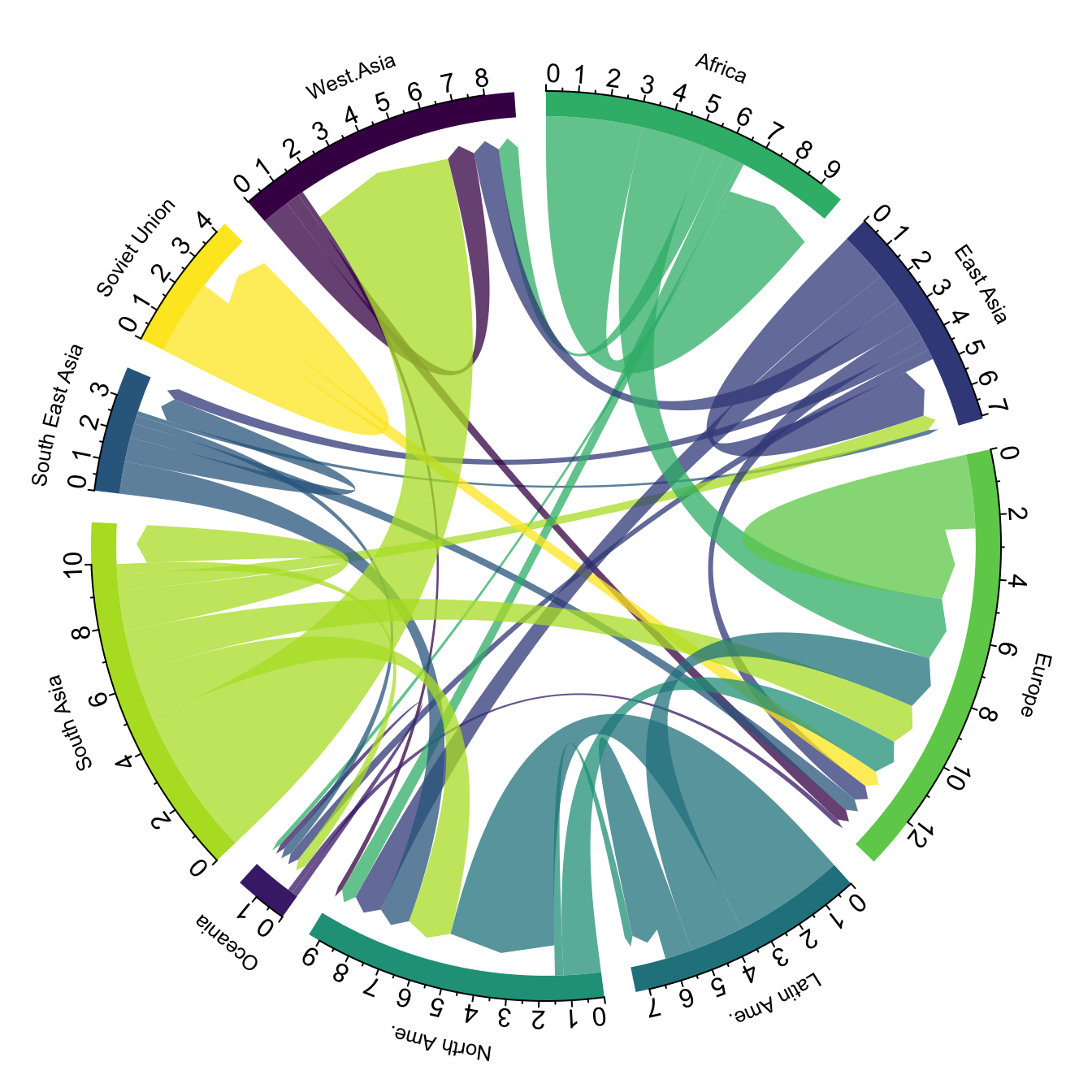# Definition

A `chord diagram` represents flows or connections between several entities (called `nodes`). Each entity is represented by a fragment on the outer part of the `circular layout`. Then, `arcs` are drawn between each entities. The size of the arc is proportional to the importance of the flow.

Here is an example displaying the number of people migrating from one country to another. Data used comes from this scientific publication from Gui J. Abel.

``````# Libraries
library(tidyverse)
library(viridis)
library(patchwork)
library(hrbrthemes)
library(circlize)
library(chorddiag)  #devtools::install_github("mattflor/chorddiag")

# short names
colnames(data) <- c("Africa", "East Asia", "Europe", "Latin Ame.",   "North Ame.",   "Oceania", "South Asia", "South East Asia", "Soviet Union", "West.Asia")
rownames(data) <- colnames(data)

# I need a long format
data_long <- data %>%
rownames_to_column %>%
gather(key = 'key', value = 'value', -rowname)

# parameters
circos.clear()
circos.par(start.degree = 90, gap.degree = 4, track.margin = c(-0.1, 0.1), points.overflow.warning = FALSE)
par(mar = rep(0, 4))

# color palette
mycolor <- viridis(10, alpha = 1, begin = 0, end = 1, option = "D")
mycolor <- mycolor[sample(1:10)]

# Base plot
chordDiagram(
x = data_long,
grid.col = mycolor,
transparency = 0.25,
directional = 1,
direction.type = c("arrows", "diffHeight"),
diffHeight  = -0.04,
annotationTrack = "grid",
annotationTrackHeight = c(0.05, 0.1),

circos.trackPlotRegion(
track.index = 1,
bg.border = NA,
panel.fun = function(x, y) {

xlim = get.cell.meta.data("xlim")
sector.index = get.cell.meta.data("sector.index")

# Add names to the sector.
circos.text(
x = mean(xlim),
y = 3.2,
labels = sector.index,
facing = "bending",
cex = 0.8
)

circos.axis(
h = "top",
major.at = seq(from = 0, to = xlim, by = ifelse(test = xlim>10, yes = 2, no = 1)),
minor.ticks = 1,
major.tick.percentage = 0.5,
labels.niceFacing = FALSE)
}
)``````# What for

Chord diagrams are eye catching and quite popular in data visualization. They allow to visualize `weigthed` relationships between several entities. They are adapted for several specific situations that slightly modify the output and the way to read them:

• Flow. This is the example decribed in the chord diagram above. But two ways to represent it:

• One asymetric arc per pair
• Two arcs per pair
• Bipartite: nodes are grouped in a few categories. Connections go between categories but not within categories. In my opinion sankey diagrams are more adapted in this situation.

Note: this section is under construction.

# Variation

Interactivity is a real plus to make the chord diagram understandable. In the example below, you can hover a specific group to highlight all its connections.

``````library(chorddiag)
m <- matrix(c(11975,  5871, 8916, 2868,
1951, 10048, 2060, 6171,
8010, 16145, 8090, 8045,
1013,   990,  940, 6907),
byrow = TRUE,
nrow = 4, ncol = 4)
haircolors <- c("black", "blonde", "brown", "red")
dimnames(m) <- list(have = haircolors,
prefer = haircolors)

groupColors <- c("#000000", "#FFDD89", "#957244", "#F26223")
chorddiag(m, groupColors = groupColors, groupnamePadding = 20)``````

Note: this example comes from the chorddiag package documentation.

# Common mistakes

• The group order around the circle is important. Try to minimize the number of arc crossing.
• Mind over-cluttering that makes the figure unreadable. It is advised to dismiss weak connections.
• Chord diagrams are not straightforward to understand at all. Give plenty of explanation to your audience when you present it. It is advised to break down the graphic, presenting its components progressively. See this awesome example of storytelling from Nadieh Bremer.

# Related

The R and Python graph galleries are 2 websites providing hundreds of chart example, always providing the reproducible code. Click the button below to see how to build the chart you need with your favorite programing language.